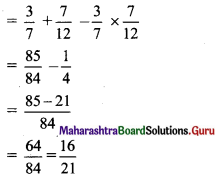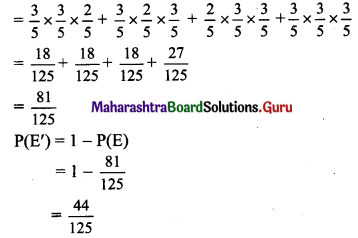# Maharashtra Board 11th Maths Solutions Chapter 9 Probability Ex 9.5

Balbharti Maharashtra State Board Class 11 Maths Solutions Pdf Chapter 9 Probability Ex 9.5 Questions and Answers.

## Maharashtra State Board 11th Maths Solutions Chapter 9 Probability Ex 9.5

Question 1.
If odds in favour of X solving a problem are 4 : 3 and odds against Y solving the same problem are 2 : 3. Find the probability of:
(i) X solving the problem
(ii) Y solving the problem
Solution:
(i) Odds in favour of X solving a problem are 4 : 3.
∴ The probability of X solving the problem is
P(X) = $$\frac{4}{4+3}=\frac{4}{7}$$

(ii) Odds against Y solving the problem are 2 : 3.
∴ The probability of Y solving the problem is
P(Y) = 1 – P(Y’)
= 1 – $$\frac{2}{2+3}$$
= 1 – $$\frac{2}{5}$$
= $$\frac{3}{5}$$Question 2.
The odds against John solving a problem are 4 to 3 and the odds in favour of Rafi solving the same problem are 7 to 5. What is the chance that the problem is solved when both of them try it?
Solution:
The odds against John solving a problem are 4 to 3.
Let event P(A’) = P (John does not solve the problem)
= $$\frac{4}{4+3}$$
= $$\frac{4}{7}$$
So, the probability that John solves the problem
P(A) = 1 – P(A’) = 1 – $$\frac{4}{7}$$ = $$\frac{3}{7}$$
Similarly, Let P(B) = P(Rafi solves the problem)
Since the odds in favour of Rafi solving the problem are 7 to 5,
P(B) = $$\frac{7}{7+5}$$ = $$\frac{7}{12}$$
Required probability
P(A ∪ B) = P(A) + P(B) – P(A ∩ B)
Since A, B are independent events,
P(A ∩ B) = P(A) . P(B)
∴ Required probability = P(A) + P(B) – P(A) . P(B)Question 3.
The odds against student X solving a statistics problem are 8 : 6 and odds in favour of student Y solving the same problem are 14 : 16. Find the chance that
(i) the problem will be solved if they try it independently.
(ii) neither of them solves the problem.
Solution:
The odds against X solving a problem are 8 : 6.
Let P(X’) = P(X does not solve the problem) = $$\frac{8}{8+6}$$ = $$\frac{8}{14}$$
So, the probability that X solves the problem
P(X) = 1 – P(X’) = 1 – $$\frac{8}{14}$$ = $$\frac{6}{14}$$
Similarly, let P(Y) = P(Y solves the problem)
Since odds in favour of Y solving the problem are 14 : 16,
P(Y) = $$\frac{14}{14+16}=\frac{14}{30}$$
So, the probability that Y does not solve the problem
P(Y’) = 1 – P(Y)
= 1 – $$\frac{14}{30}$$
= $$\frac{16}{30}$$
(i) Required probability
P(X ∪ Y) = P(X) + P(Y) – P(X ∩ Y)
Since X and Y are independent events,
P(X ∩ Y) = P(X) . P(Y)
∴ Required probability = P(X) + P(Y) – P(X) . P(Y)
= $$\frac{6}{14}+\frac{14}{30}-\frac{6}{14} \times \frac{14}{30}$$
= $$\frac{73}{105}$$

(ii) Required probability = P(X’ ∩ Y’)
Since X and Y are independent events, X’ and Y’ are also independent events.
∴ Required probability = P(X’) . P(Y’)
= $$\frac{8}{14} \times \frac{16}{30}$$
= $$\frac{32}{105}$$Question 4.
The odds against a husband who is 60 years old, living till he is 85 are 7 : 5. The odds against his wife who is now 56, living till she is 81 are 5 : 3. Find the probability that
(i) at least one of them will be alive 25 years hence.
(ii) exactly one of them will be alive 25 years hence.
Solution:
The odds against her husband living till he is 85 are 7 : 5.
Let P(H’) = P(husband dies before he is 85) = $$\frac{7}{7+5}=\frac{7}{12}$$
So, the probability that the husband would be alive till age 85
P(H) = 1 – P(H’) = 1 – $$\frac{7}{12}$$ = $$\frac{5}{12}$$
Similarly, P(W’) = P(Wife dies before she is 81)
Since the odds against wife will be alive till she is 81 are 5 : 3.
∴ P(W’) = $$\frac{5}{5+3}=\frac{5}{8}$$
So, the probability that the wife would be alive till age 81
P(W) = 1 – P(W’) = 1 – $$\frac{5}{8}$$ = $$\frac{3}{8}$$
(i) Required probability
P(H ∪ W) = P(H) + P(W) – P(H ∩ W)
Since H and W are independent events,
P(H ∩ W) = P(H) . P(W)
∴ Required probability = P(H) + P(W) – P(H) . P(W)
= $$\frac{5}{12}+\frac{3}{8}-\frac{5}{12} \times \frac{3}{8}$$
= $$\frac{40+36-15}{96}$$
= $$\frac{61}{96}$$

(ii) Required probability = P(H ∩ W’) + P(H’ ∩ W)
Since H and W are independent events, H’ and W’ are also independent events.
∴ Required probability = P(H) . P(W’) + P(H’) . P(W)
= $$\frac{5}{12} \times \frac{5}{8}+\frac{7}{12} \times \frac{3}{8}$$
= $$\frac{25+21}{96}$$
= $$\frac{46}{96}$$
= $$\frac{23}{48}$$Question 5.
There are three events A, B, and C, one of which must, and only one can happen. The odds against event A are 7 : 4 and odds against event B are 5 : 3. Find the odds against event C.
Solution:
Since odds against A are 7 : 4,
P(A) = $$\frac{4}{7+4}=\frac{4}{11}$$
Since odds against B are 5 : 3,
P(B) = $$\frac{3}{5+3}=\frac{3}{8}$$
Since only one of the events A, B and C can happen,
P(A) + P(B) + P(C) = 1
$$\frac{4}{11}$$ + $$\frac{3}{8}$$ + P(C) = 1
∴ P(C) = 1 – ($$\frac{4}{11}$$ + $$\frac{3}{8}$$)
= 1 – $$\left(\frac{32+33}{88}\right)$$
= $$\frac{23}{88}$$
∴ P(C’) = 1 – P(C)
= 1 – $$\frac{23}{88}$$
= $$\frac{65}{88}$$
∴ Odds against the event C are P(C’) : P(C)
= $$\frac{65}{88}$$ : $$\frac{23}{88}$$
= 65 : 23

Question 6.
In a single toss of a fair die, what are the odds against the event that number 3 or 4 turns up?
Solution:
When a fair die is tossed, the sample space is
S = {1, 2, 3, 4, 5, 6}
∴ n(S) = 6
Let event A: 3 or 4 turns up.
∴ A = {3, 4}
∴ n(A) = 2
∴ P(A) = $$\frac{n(A)}{n(S)}$$ = $$\frac{2}{6}=\frac{1}{3}$$
P(A’) = 1 – P(A) = 1 – $$\frac{1}{3}$$ = $$\frac{2}{3}$$
∴ Odds against the event A are P(A’) : P(A)
= $$\frac{2}{3}: \frac{1}{3}$$
= 2 : 1Question 7.
The odds in favour of A winning a game of chess against B are 3 : 2. If three games are to be played, what are the odds in favour of A’s winning at least two games out of the three?
Solution:
Let event A: A wins the game and event B: B wins the game.
Since the odds in favour of A winning a game against B are 3 : 2,
the probability of occurrence of event A and B is given by
P(A) = $$\frac{3}{3+2}=\frac{3}{5}$$ and P(B) = $$\frac{2}{3+2}=\frac{2}{5}$$
Let event E: A wins at least two games out of three games.
∴ P(E) = P(A) . P(A) . P(B) + P(A) . P(B) . P(A) + P(B) . P(A) . P(A) + P(A) . P(A) . P(A)∴ Odds in favour of A’s winning at least two games out of three are P(E) : P(E’)
= $$\frac{81}{125}: \frac{44}{125}$$
= 81 : 44